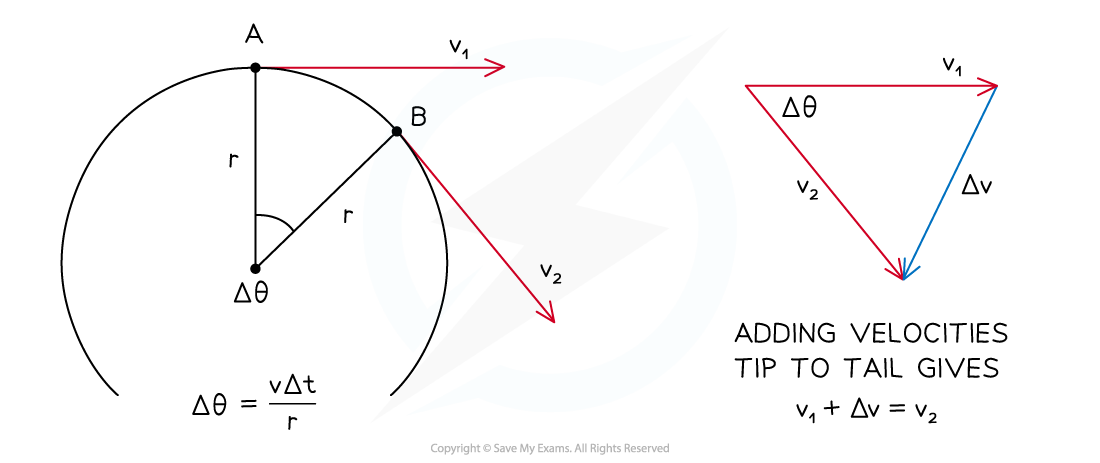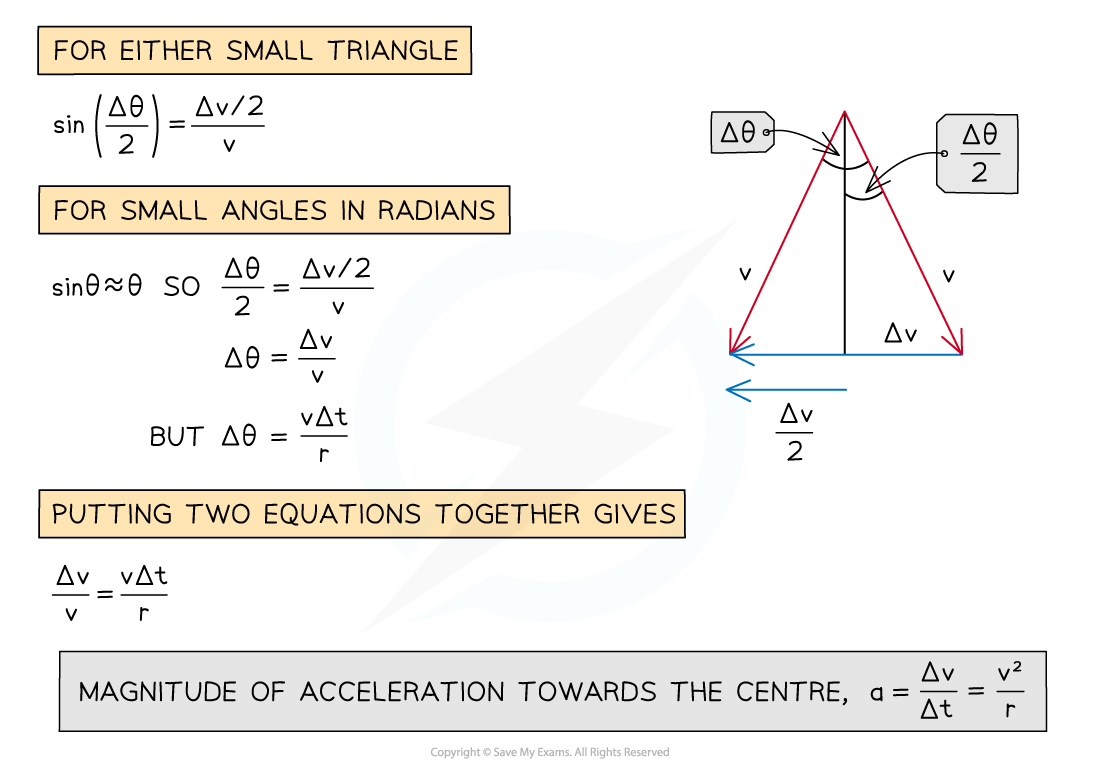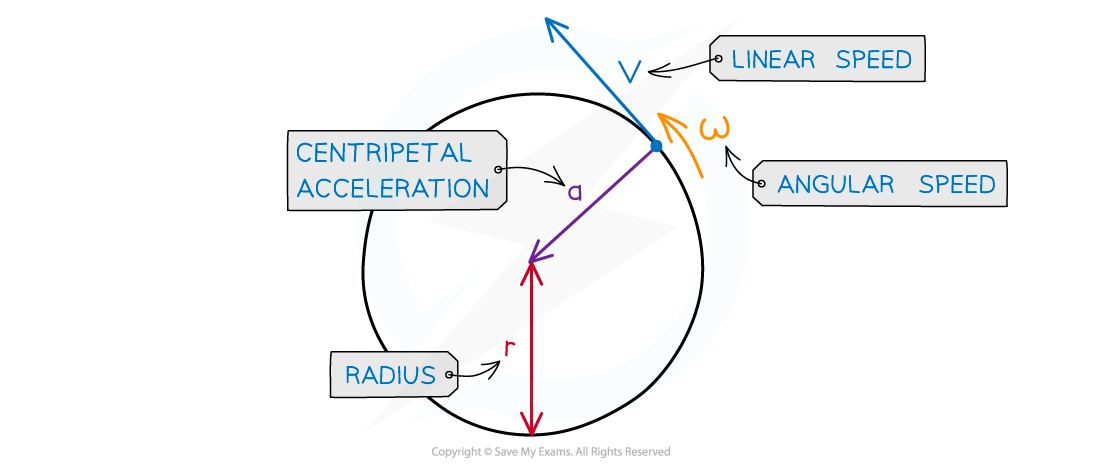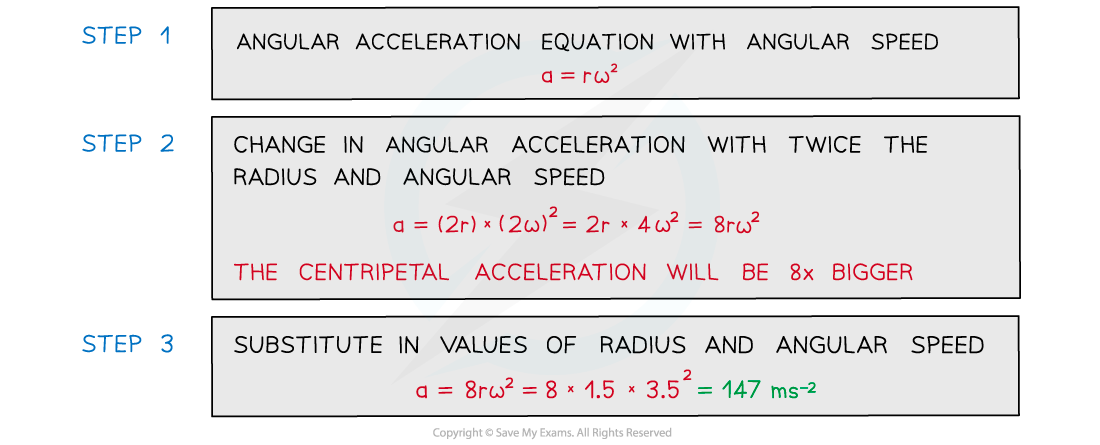# IB DP Physics: HL复习笔记6.1.3 Centripetal Acceleration

### Centripetal Acceleration

• For an object moving in a circle:
• The acceleration is towards the centre of the circle
• The magnitude of the centripetal acceleration a is:• Where:
• a = centripetal acceleration (m s–2)
• v = linear speed (m s–1)
• r = radius of orbit (m)
• Uniform circular motion is continuously changing direction, and therefore is constantly changing velocity
• The object must therefore be accelerating
• This is called the centripetal acceleration

#### Direction of the Centripetal Acceleration

• The centripetal acceleration is perpendicular to the direction of the linear velocity
• Centripetal means it acts towards the centre of the circular pathSlide a ruler parallel to Δv towards the circle. Midway between A and B, Δv points towards the centre of the circle. This is the same direction as the centripetal acceleration

• If an object moves through a section of a circle during some time Δt
• The change in velocity during this time is Δv
• The centripetal acceleration is Δv (a vector) divided by Δt (a scalar)
• The centripetal acceleration points in the same direction as the change in velocity Δv
• The centripetal acceleration is caused by a centripetal force of constant magnitude that also acts perpendicular to the direction of motion (towards the centre)
• There is no component of the centripetal force in the direction of the velocity
• Therefore, there is no acceleration in the direction of the velocity
• Hence, there is uniform motion at constant speed
• Therefore, the centripetal acceleration and force act in the same direction

#### Magnitude of the Centripetal Acceleration

• In the diagram above notice how the angle Δθ is defined in terms of the arc length vΔt and the radius r
• v is the magnitude of v1 and v2
• Notes for deriving the equation for centripetal acceleration:
• The vector triangle should be formed so that Δv is horizontal
• The velocity vectors v should be of the same length
• Hence, the vertical line bisects the angle Δθ and the vector Δv
• Use trigonometry for one of the small triangles
• The small-angle approximation requires that the angles are in radians
• The two equations for Δθ lead to the magnitude of the centripetal accelerationDeriving the equation for the magnitude of the centripetal acceleration

• This leads to the equation for centripetal acceleration:• Using the equation relating angular speed ω and linear speed v:

v = r⍵

• These equations can be combined to give another form of the centripetal acceleration equation:• Where:
• a = centripetal acceleration (m s2)
• v = linear speed (m s1)
• ⍵ = angular speed (rad s1)
• r = radius of the orbit (m)
• Uniform centripetal acceleration is defined as:

The acceleration of an object towards the centre of a circle when an object is in motion (rotating) around a circle at a constant speedCentripetal acceleration is always directed toward the centre of the circle and is perpendicular to the object’s velocity

#### Worked Example

A domestic washing machine has a spin cycle of 1200 rpm (revolutions per minute) and a diameter of 50 cm.

Calculate the centripetal acceleration experienced by the washing during the spin cycle.

Step 1: List the known quantities

• Radius of the drum, r = ½ × 50 cm = 25 cm

Step 2: Convert the revolutions per minute to revolutions per second

1200 ÷ 60 = 20 rev s−1

Step 3: Convert revolutions per second to angular speed in radians per second

1 rev s–1 = 2π rad s–1

20 rev s–1 = 40π rad s–1 = ω

Step 4: Write the equation linking centripetal acceleration and angular speed

a = 2

Step 5: Calculate the centripetal acceleration

a = (25 × 10−2) × (40π)2

Step 6: State the final answer

a = 3900 m s−2 (2 s.f.)

#### Worked Example

A ball tied to a string is rotating in a horizontal circle with a radius of 1.5 m and an angular speed of 3.5 rad s−1.

Calculate its centripetal acceleration if the radius was twice as large and angular speed was twice as fast.#### Exam Tip

The key takeaways for an object moving in a circle are:

• The magnitude of the velocity vector does not change
• The direction of the velocity vector does change
• Therefore, there is an acceleration despite the speed not changing